Mobile QR Code1. (Department of Electrical and Computer Engineering, Seoul National University, Seoul 08826, Korea)
2. (SK Hynix, Ichoen 17336, Korea)

Quadrature error corrector, phase mismatches, data strobe, HBM3

## I. INTRODUCTION

The emerging high bandwidth memory generation 3 (HBM3) interfaces require a quadrature error corrector (QEC) that ensures the uniform spacings among the quadrature phases of the data strobe (DQS) signal. The ever-increasing demand for the higher data rates in HBM interfaces  led to the increase in the data (DQ) and data strobe (DQS) signal frequencies, which tightened the requirement for their timing skews. Previously, it was sufficient just to correct the phase spacings of the quadrature clocks sent by the memory. Now, it is also necessary to correct the phase spacings of the quadrature DQS signals, propagating through through-silicon vias (TSVs) and buffers. This paper presents a QEC circuit that can eliminate the phase imbalance in quadrature DQS signals.

Fig. 1 illustrates the timing diagram of the HBM3 clocking. The HBM3 device receives 1.6-GHz differential clock inputs (CK_t/CK_c) and 3.2-GHz differential write strobe inputs (WDQS_t/WDQS_c) during write operations . To alleviate the timing constraints of the internal circuits, the WDQS signal is divided down to 4 quarter-rate WDQS/2 signals, each with the phase of 0, 90, 180, and 270$^{\circ}$, respectively. Our goal is to maintain uniform phase spacings among these WDQS/2 signals.

Several approaches have been proposed to address the phase imbalance in quadrature signals, including the use of a shared feedback structure with digitally controlled delay lines [3,4], a relaxation oscillator-based phase detector , and replica serializers and pulse-shrinking delay lines . However, these approaches are only capable of correcting the phase imbalance in periodic clock signals since they all rely on the arrival of the next-period pulses. They are not applicable to correcting the DQS signals operating in a burst mode, where such periodicity is not guaranteed.

##### Fig. 1. The timing diagram of HBM3 clocking.}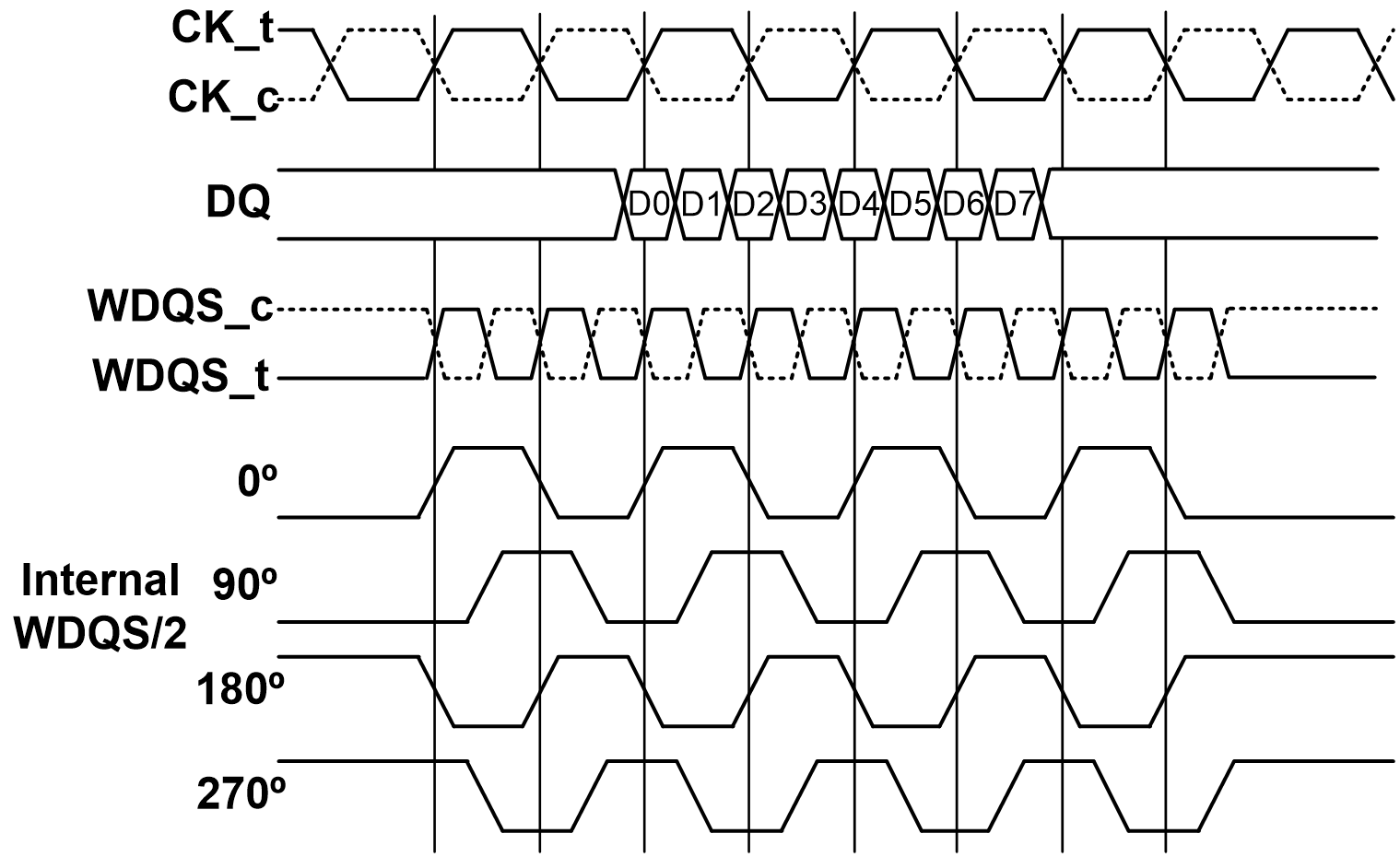To correct the skew of the DQS signals, the proposed QEC compares each phase interval of the DQS signals to 1/4 of the clock period. The DQS phase error is detected by charging a capacitor with a fixed current during the phase interval and comparing its voltage against the voltage of a four-times larger capacitor charged for the entire clock period. The detected error is then corrected by adjusting the delay of the delay lines propagating the quadrature DQS signals. The QEC can be turned off while fixing the delays, to reduce power consumption after the calibration is complete. The post-layout simulation results demonstrate a correctable input phase error range of -43.2~43.2˚ and a maximum post-correction phase error of 5.01˚, while consuming a maximum power of 2.42 mW at a frequency of 1.6 GHz.

The rest of this paper is organized as follows. Section II describes the operating principle and the overall system architecture of the proposed QEC. Section III presents the circuit implementation of the proposed QEC. Finally, Section IV and V present the simulation results based on the post-layout netlist and the conclusion, respectively.

## II. SYSTEM ARCHITECTURE

The proposed QEC operates in two modes—CLK mode and DQS mode. A simplified diagram and the operation of the main block in the two modes are depicted in Fig. 2. The main block comprises a current digital-to-analog converter (IDAC), capacitors, and a comparator. The pulse with a pulse-width equal to the period of the clock signal, T$_{clk}$, is denoted by clk_pulse and the pulse with a pulse-width equal to the phase difference between two adjacent DQS signals, T$_{dqs}$, is denoted by dqs_pulse. In the figure, V$_{C}$ denotes the voltage of the capacitor, d denotes the output of the comparator, and V$_{th,comp}$ denotes the threshold voltage of the comparator. During the CLK mode, the QEC stores the period information of the clock signal in the IDAC control codes while charging four identical capacitors for T$_{clk}$. The IDAC control code is adjusted to make the post-charging value of V$_{C}$ equal to V$_{th,comp}$. Next, in the DQS mode, the time difference between the adjacent DQS signals is adjusted to 1/4 of the clock period. One of the four capacitors is charged for T$_{dqs}$. T$_{dqs}$ is adjusted to make the post-charging value of V$_{C}$ equal to V$_{th,comp}$. As the capacitance ratio is taken to be 4:1, T$_{dqs}$ becomes 1/4 of T$_{clk}$. Therefore, it is possible to correct the phase imbalance in aperiodic signals by exploiting the presence of the same-period clock signal. As the proposed method stores the period information, it can operate correctly irrespective of the duty cycle of the clock signal.

##### Fig. 2. Two modes of the proposed quadrature error corrector.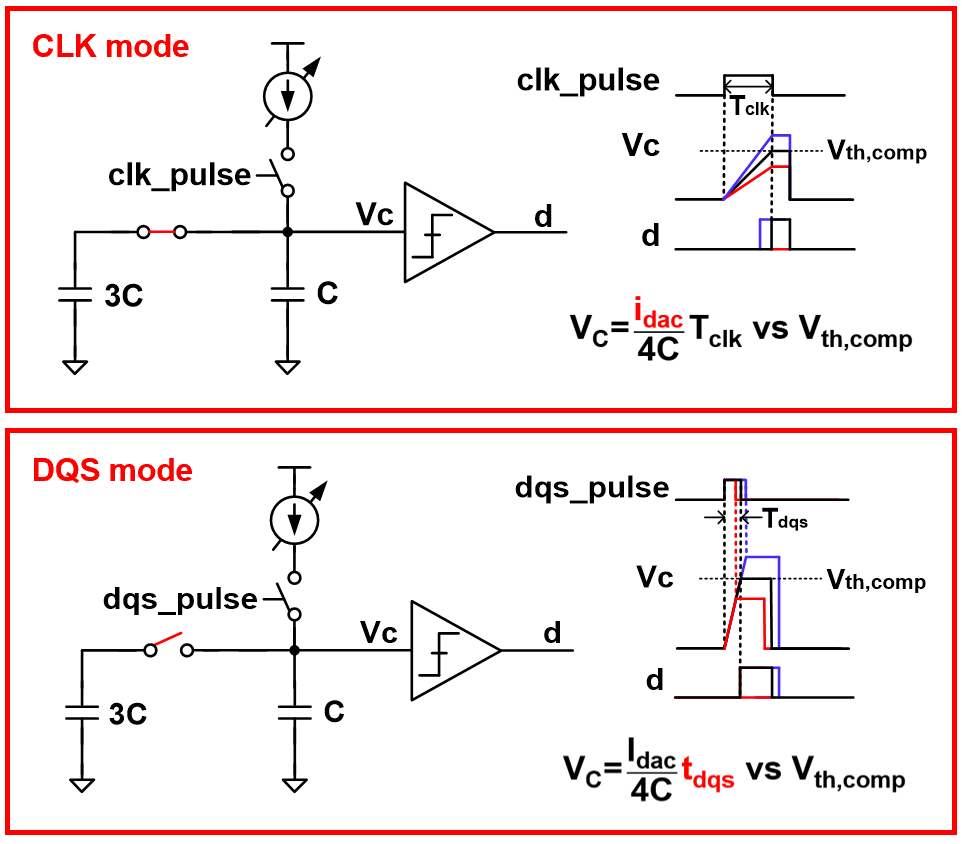Fig. 3 illustrates the overall block diagram of the proposed QEC, comprising the main path and the feedback loop. The main path is composed of one delay line with a fixed delay time for the signal, I, and three 8-bit digitally controlled delay lines (DCDLs) to adjust the delays of the signals, Q, IB, and QB. The feedback loop consisted of a pulse generator, a pulse-width detector in charge of the main block of the QEC, a DIV8&3bit-counter, and a digital loop filter. The operation is as follows. The pulse generator alternately generates a pulse with a pulse-width equal to the clock period (T$_{clk}$) or the phase difference between neighboring DQS signals (T$_{dqs}$). The pulse-width detector stores the clock period information in the IDAC control codes using a T$_{clk}$-wide pulse in the CLK mode. In the DQS mode, the pulse-width detector outputs a 1-bit digital signal, which compares phase difference of the DQS signals with 1/4 of the clock period using the stored information about the clock period. To reduce the power consumption of the digital loop filter, the DIV8 block creates a clock for the digital loop filter, which operates at up to 1/8 of the clock frequency. Additionally, the 3bit-counter is used to count the 1-bit output of the pulse-width detector seven times and converts it to a 3-bit output. The digital loop filter is used to control the IDAC control codes in the pulse-width detector to save the clock period information in the CLK mode. In the DQS mode, the DCDL control codes are controlled by the digital loop filter using the majority vote method to adjust the delays of the quadrature signals.

##### Fig. 3. Block diagram of the proposed quadrature error corrector.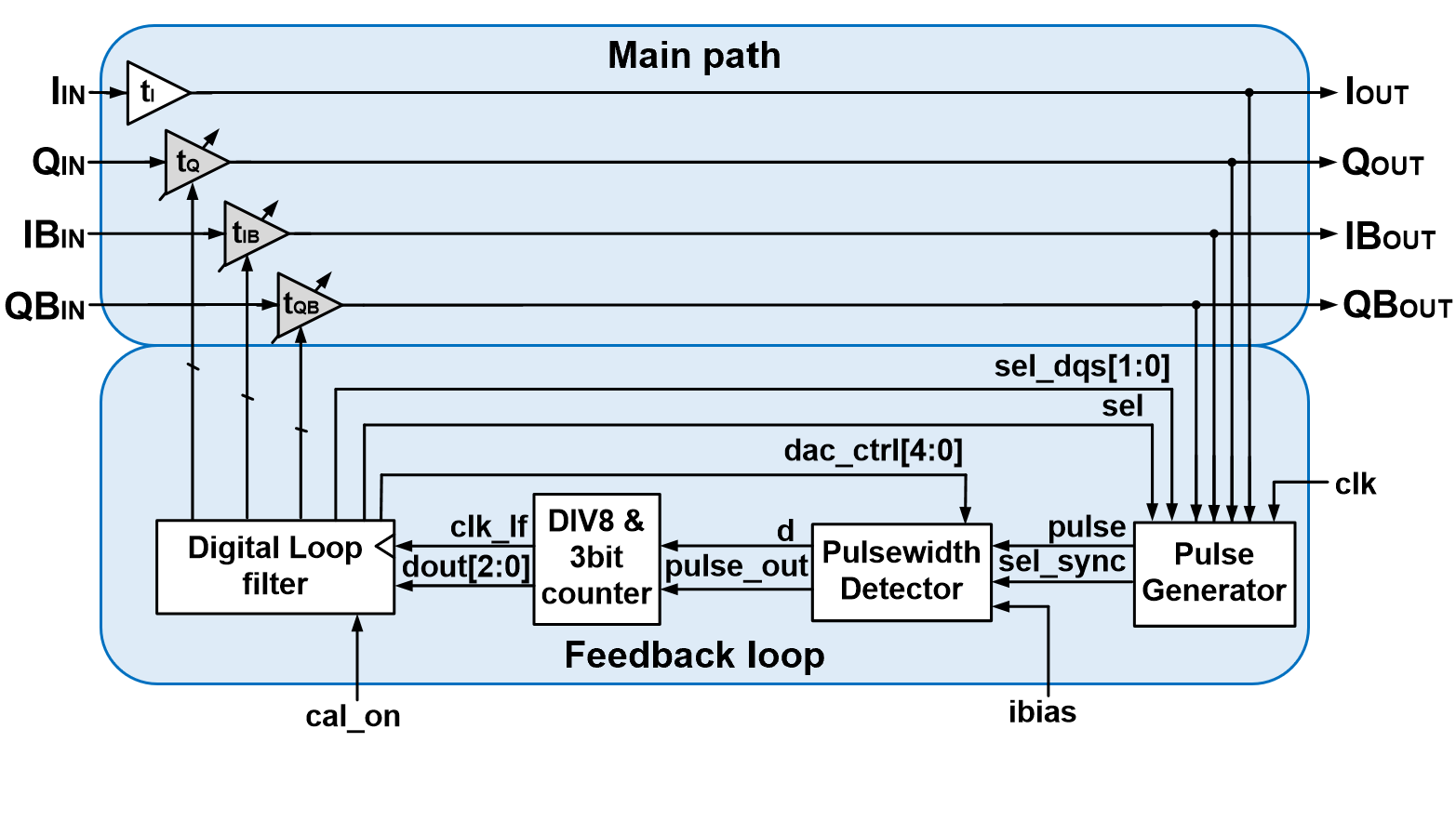After the calibration, the QEC can be turned off to reduce the power consumption while only activating the DCDLs with fixed delays . When an external signal, cal_on becomes low, all the DCDL control codes are fixed and the feedback loop is stopped. The calibration can be re-activated periodically by re-asserting cal_on high to compensate the change in delays due to process, voltage, or temperature variations.

## III. CIRCUIT DESCRIPTION

### 1. Pulse-width Detector

The main block is a pulse-width detector that detects the 1/4 point of the clock period. The pulse-width detector operates in three modes, as depicted in Fig. 4. There are CLK mode and DQS mode as mentioned in Section II. When neither mode is active, the block is set to be in REST mode. The pulse-width detector comprises an IDAC, four identical capacitors, and a comparator. In the CLK mode, the four capacitors are charged for the time equal to the clock period, and a 1-bit output is obtained by comparing the threshold voltage of the comparator and the voltage of the capacitors, V$_{C}$, which depends on the amount of current. In the DQS mode, only one capacitor is charged with the fixed IDAC control codes, and, as in the CLK mode, a 1-bit output is obtained by comparing the threshold voltage of the comparator and the voltage of the capacitor, V$_{C}$, which depends on the charging time, the phase difference between neighboring DQS signals. The ratio of the capacitances in the CLK and DQS modes is 4:1—therefore, the phase difference of the DQS signals is adjusted to be equal to 1/4 of the clock period. In the REST mode, the current is diverted along another path to reduce error due to charge sharing, as explained in the following paragraph.

##### Fig. 4. Three modes of pulse-width detector.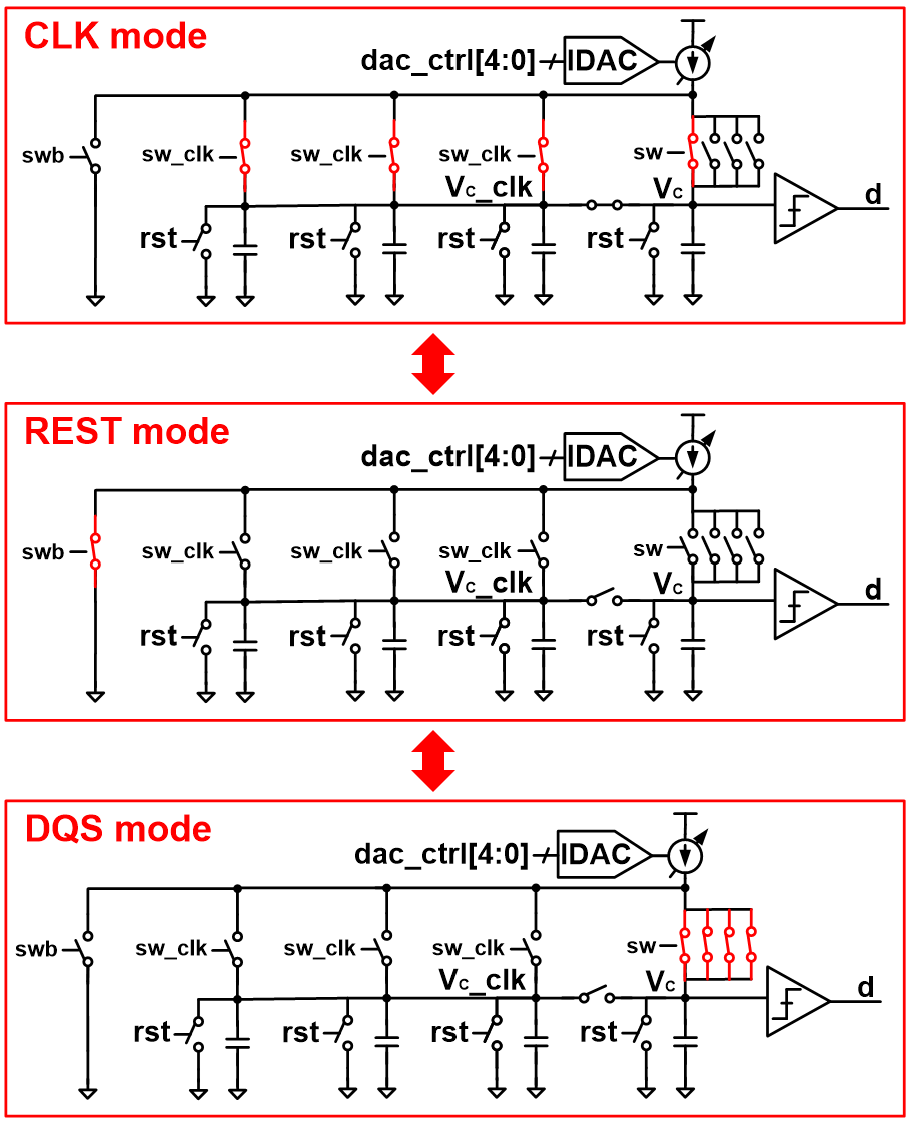An important design issue is that the initial voltage and the amount of current flowing in the CLK and DQS modes must be the same. The detailed implementation of the pulse-width detector is depicted in Fig. 5(a). When the switches (M$_{\mathrm{S2}}$, M$_{\mathrm{S3}}$, M$_{\mathrm{S4}}$, M$_{\mathrm{S5}}$, M$_{\mathrm{S6}}$, M$_{\mathrm{S7}}$, or M$_{\mathrm{S8}}$) turn on to charge the capacitors, the initial voltage may not be at zero due to a charge sharing with node A. The effect of charge sharing can be reduced by keeping the voltage on node A constant. In the absence of any current, the voltage of node A becomes equal to the supply voltage. Therefore, in the REST mode, the voltage of node A is maintained constant by diverting the current along another path, as illustrated in Fig. 4. The switch logic depicted in Fig. 5(b) creates switch pulses for switches in the pulse-width detector upon the arrival of the input pulse. As illustrated in Fig. 5(c), the IDAC uses a cascode current mirror to improve the current mirror output resistance. The offset current is always flowing through M$_{7}$ and M$_{8}$ and the amount of the current is controlled using digital control codes. The main current mirror connected to the main current source of the pulse-width detector (M$_{11}$ and M$_{12}$) uses a wide-swing current mirror with good accuracy and a high output swing.

##### Fig. 5. Detailed circuit of (a) pulse-width detector; (b) switch logic; (c) IDAC.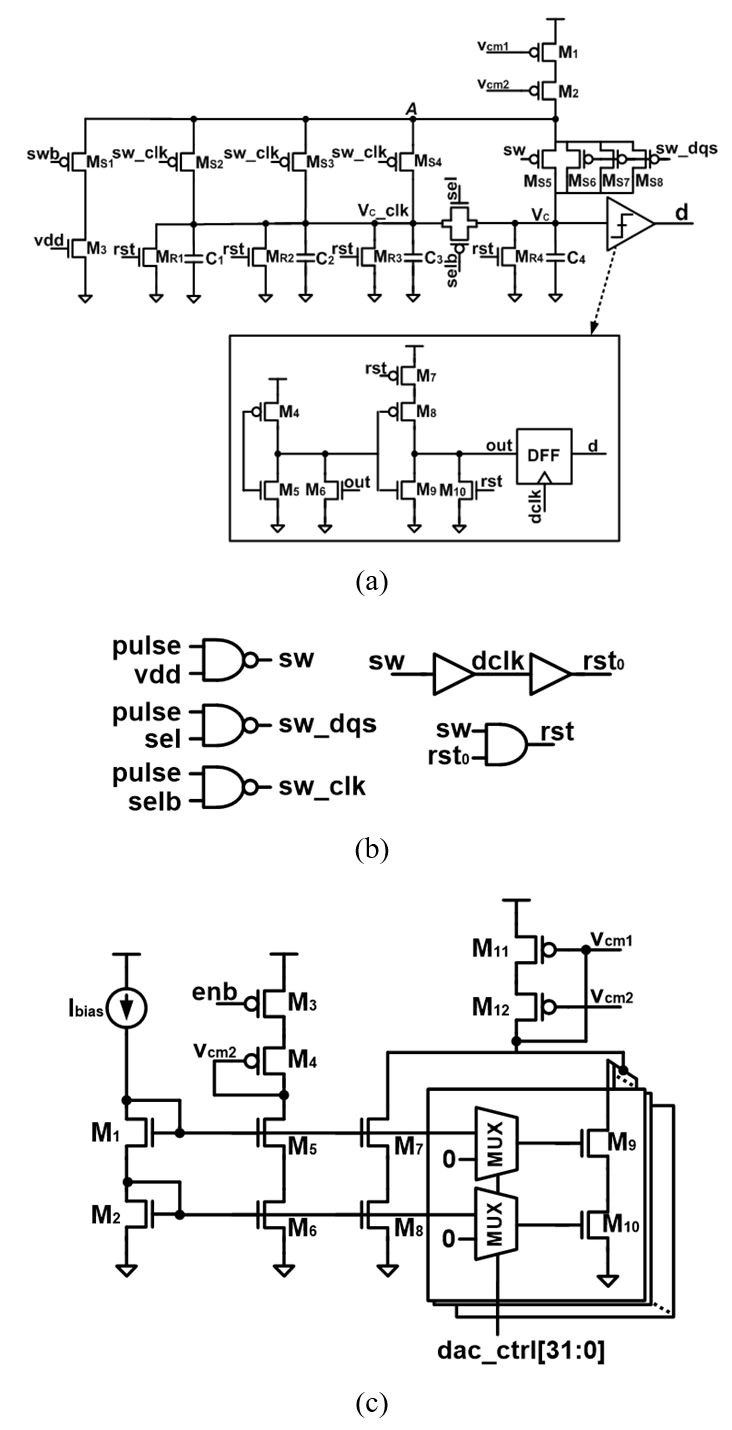To make the currents in the CLK and DQS modes equal, the number of switches turned on in the CLK and DQS modes should be equal in consideration of the transistors' channel-length modulation effects. Fig. 6 depicts a simplified circuit of the main part of the pulse-width detector, which comprises a current mirror, a switch, and a capacitor. At the beginning of charging, the switch operates in the saturation mode. The current flowing into the capacitor is given by Eqs. (1) and (2).

##### (1)
$I_{C}=\frac{1}{2}\mu _{p}C_{ox}\left(\frac{W}{L}\right)_{cm}\left(V_{DD}-V_{cm}-V_{th,p}\right)^{2}\left(1+\lambda \left(V_{DD}-V_{A}\right)\right)$.
##### (2)
$I_{C}=\frac{1}{2}\mu _{p}C_{ox}\left(\frac{W}{L}\right)_{sw}\left(V_{A}-V_{sw}-V_{th,p}\right)^{2}\left(1+\lambda \left(V_{A}-V_{C}\right)\right)$.

Eq. (1) expresses the current in terms of the current mirror, and Eq. (2) does so in terms of the switch. V$_{th,p}$ denotes the threshold voltage of the PMOS. As expressed in Eq. (1), which is also known as channel length modulation, the current is affected by the drain voltage of the current mirror, V$_{A}$. As the two values of the current expressed in Eqs. (1) and (2) are equal and the other parameters are constant, it is clear that V$_{A}$ is affected by (W/L)$_{sw}$ and the voltage of the charging capacitor, V$_{C}$. In other words, the current flowing into the capacitor is determined by (W/L)$_{sw}$ and the voltage of the charging capacitor, V$_{C}$. Therefore, to ensure that the charging currents corresponding to the same capacitor voltage are equal in both modes, the width and length ratios of the switches must be equal as well. Using an equal number of switches activated in the two modes, the currents flowing in the CLK and DQS modes can be made equal.

##### Fig. 6. Simplified circuit of main part in the pulse-width detector.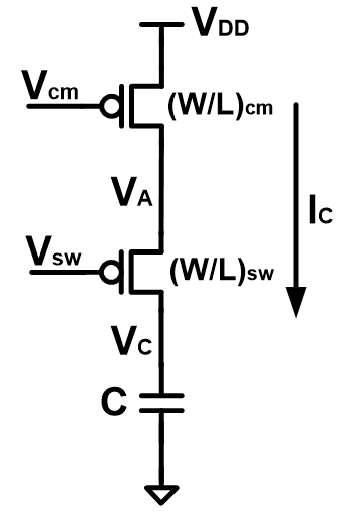A Monte-Carlo simulation of the pulse-width detector is performed using the post-layout netlist. In the CLK mode, the capacitor is charged for the period of the clock signal. In the DQS mode, the capacitor is charged for a one-quarter of the period of the clock signal. To observe the effect of the number of switches on performance, one simulation is performed with one activated switch in DQS mode, as depicted in Fig. 7(a), while the other simulation is performed with four activated switches in the DQS mode, as depicted in Fig. 7(c). The differences in the capacitor voltage, Vc, between the CLK and DQS modes over 200 sweeps are plotted in Fig. 7(b) and (d). In the ideal situation, the voltage difference would be zero. When one switch is activated in the DQS mode, the average voltage difference is 35.38 mV with a standard deviation of 5.23 mV. On the other hand, when four switches are activated in the DQS mode, the average voltage difference is -4.37 mV with a standard deviation of 4.2 mV. The better matching is achieved with activating all the four switches in both modes.

##### Fig. 7. The schematic and the histogram of capacitor voltage difference in CLK and DQS mode when using (a), (b) one switch and (c), (d) four switches.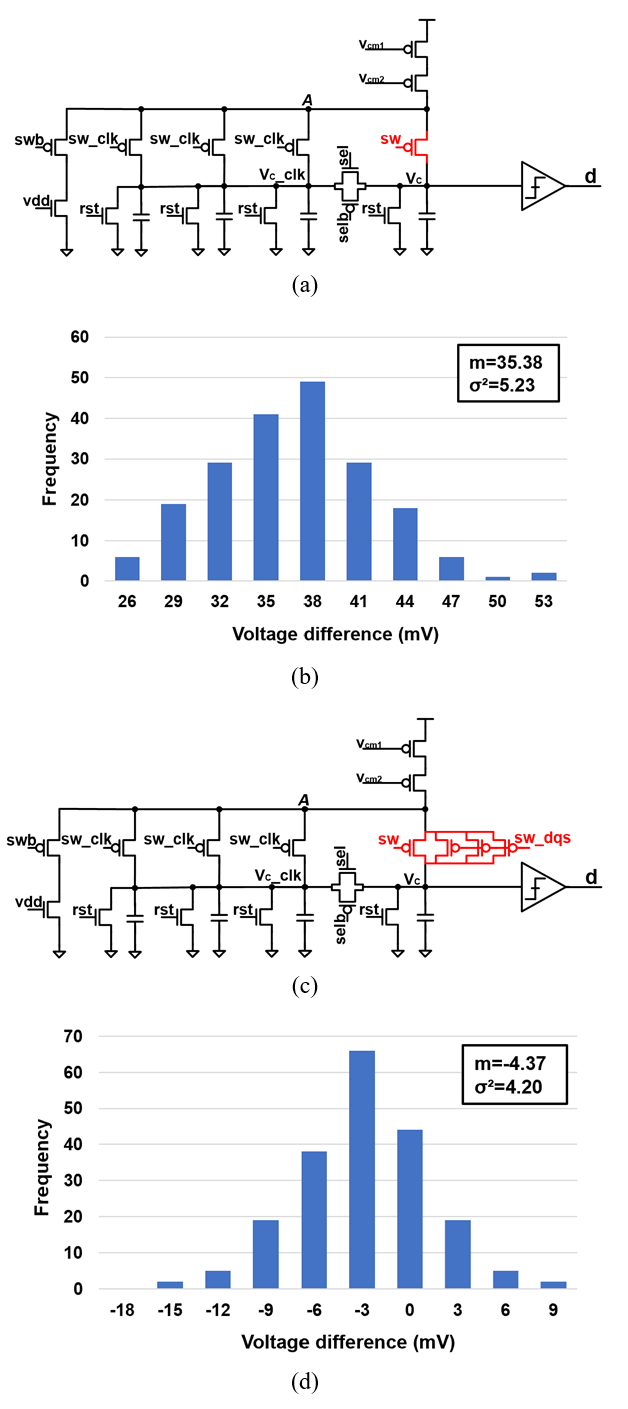### 2. Pulse Generator

The structure of the pulse generator is depicted in Fig. 8. The pulse generator consists of a CLK pulse generator, a DQS pulse generator, and a glitch-free circuit. The CLK pulse generator consists of a divide-by-two circuit and the DQS pulse generator consists of two MUXs and logic gates. In the CLK pulse generator, the clock signal is divided by two to generate clk_pulse, whose pulse-width is equal to the period of the clock signal. In the DQS pulse generator, a dqs_pulse with pulse-width equal to T$_{dqs}$ is generated. Two adjacent DQS signals are selected from each MUX and dqs_pulse is generated from the logic gates using the selected signals. Since the delay of the inverter is added to the path of one DQS signal, a transmission gate is added to the path of the other DQS signal to match the delay. The clk_pulse is selected in the CLK mode, and dqs_pulse is selected in the DQS mode as the output of the pulse generator. As illustrated by the upper waveform in Fig. 8(d), a glitch can occur during transitions between the CLK and DQS modes. Thus, the glitch-free circuit depicted in Fig. 8(c) is introduced to generate a single output between clk_pulse and dqs_pulse without a glitch. As illustrated by the lower waveform in Fig. 8(d), when the selection signal (sel) becomes high, the glitch-free circuit outputs dqs_pulse after the negative edge of clk_pulse and dqs_pulse.

##### Fig. 8. Block diagram of (a) the CLK pulse generator; (b) the DQS pulse generator; (c) the glitch-free circuit; (d) timing chart of glitch.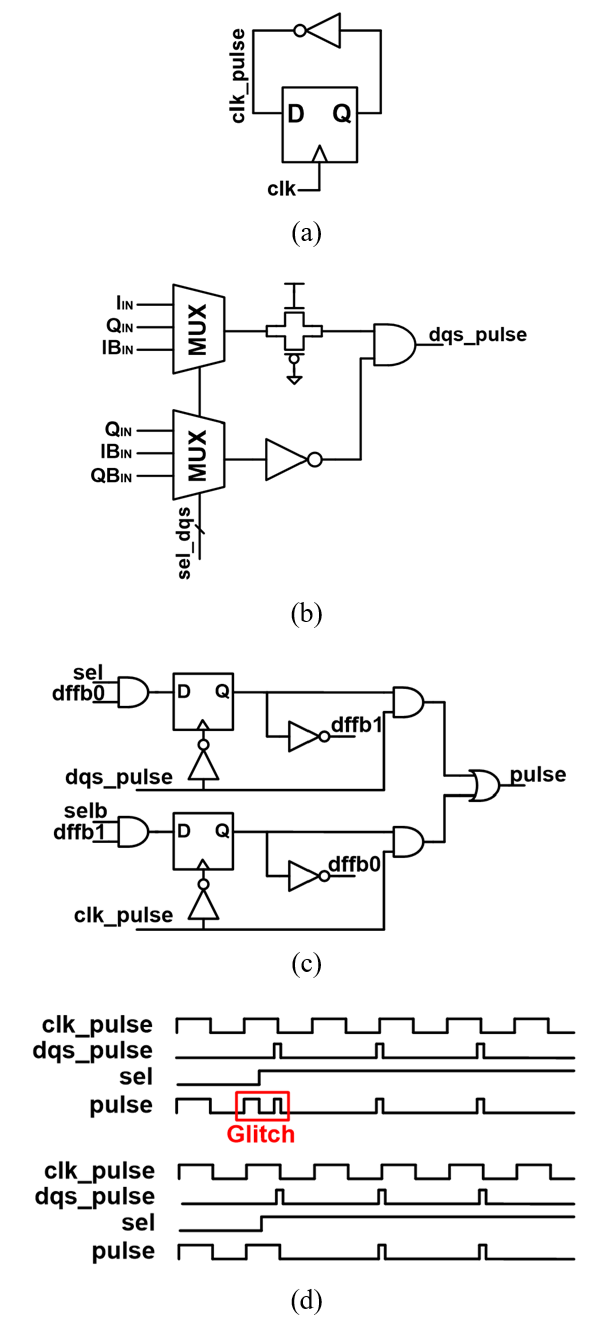### 3. Digitally Controlled Delay Lines (DCDL)

Fig. 9 presents a block diagram of the DCDL. To cover a wide error correction range, the DCDL employs NAND chain coarse delay lines merged with MOSCAP fine delay lines. The coarse and fine delay lines collectively use a 15-bit thermometer code. In coarse delay lines, a NAND chain and a MUX are used. A NAND chain, rather than an inverter chain, is used to turn off the rear end of the signal selected from the MUX. The Monte-Carlo simulation results with post-layout netlists show that the resolution and delay range of the DCDL is 0.91 ps and 153 ps, respectively.

##### Fig. 9. Block diagram of DCDL.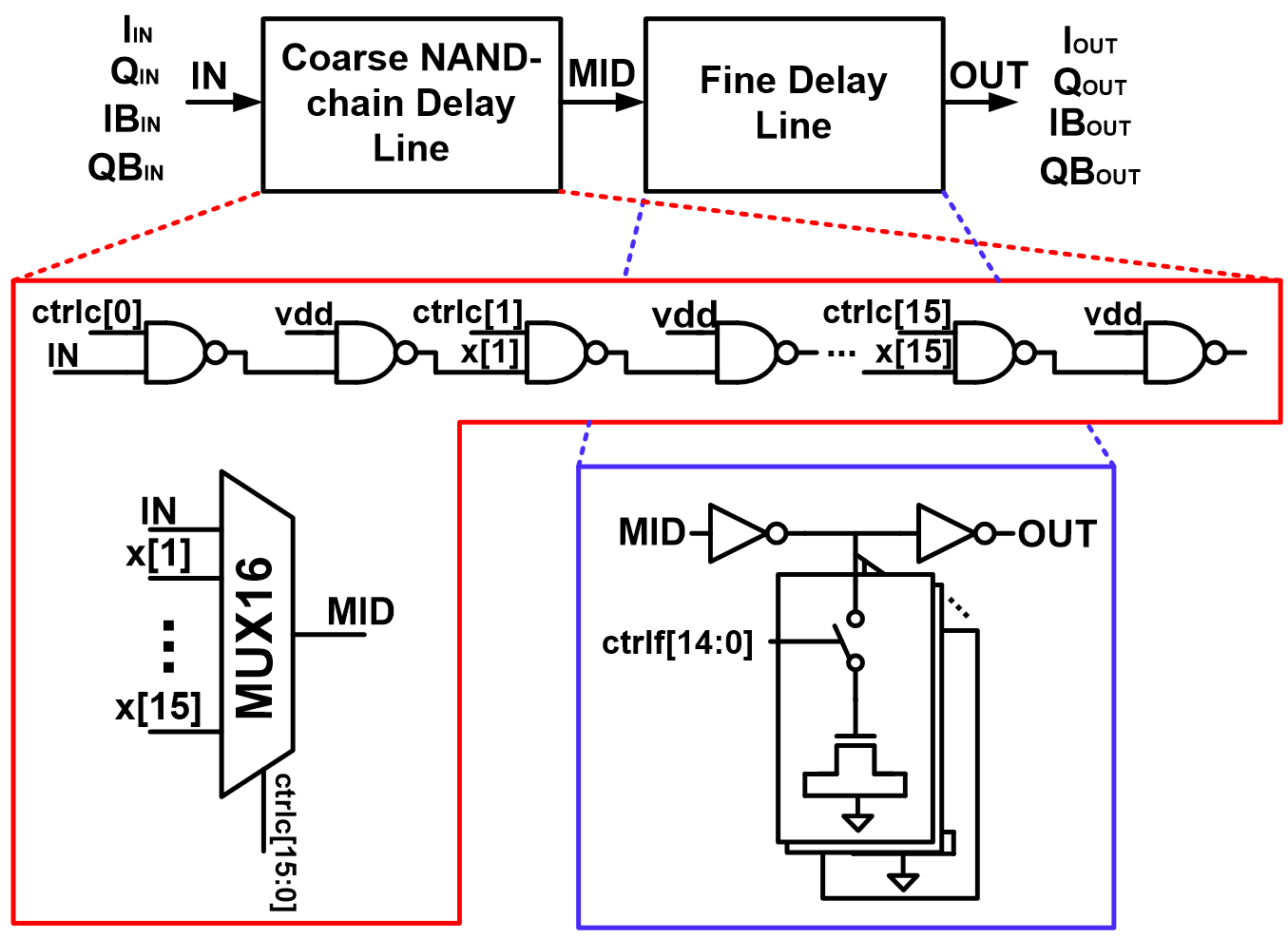### 4. DIV8 & 3-bit Counter

To reduce digital power, clk_lf, a divided clock signal produced by the DIV8 block depicted in Fig. 10(a) is used as a clock for the digital loop filter, and the 1-bit output of the pulse-width detector is accumulated into 3-bit data using a 3-bit counter, as depicted in Fig. 10(b). The DIV8 block generates clk_lf by dividing the input pulse which is clk_pulse in the CLK mode and dqs_pulse in the DQS mode by 8. The 3-bit counter counts the output of the pulse-width detector seven times and transmits a 3-bit output to the digital loop filter. Fig. 10(c) presents the timing diagram of DIV8 and the 3-bit counter. The reset signal of the 3-bit counter, rst_d, is generated by the DIV8 block. The 3-bit output data is synchronized to the negative edge of the input pulse to avoid setup and hold time violations with clk_lf.

##### Fig. 10. Block diagram of (a) DIV8 block; (b) 3-bit counter; (c) timing diagram.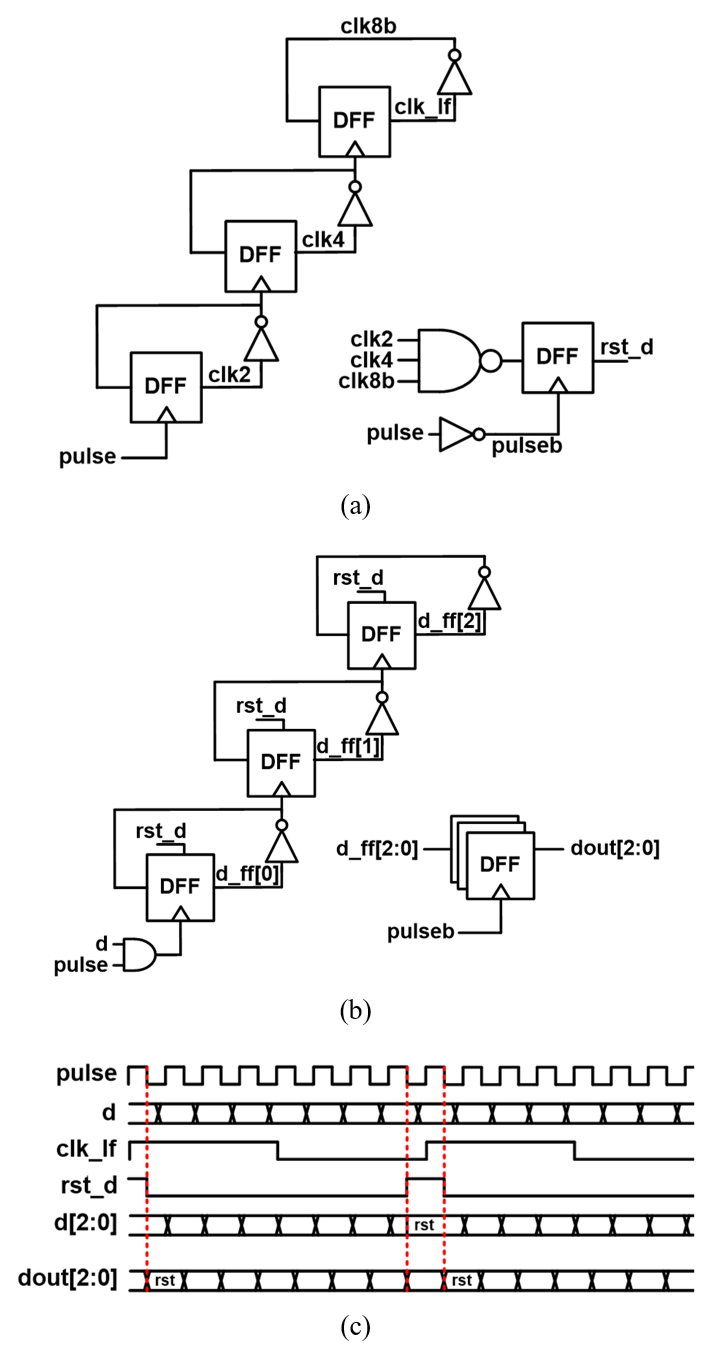### 5. Digital Loop Filter

The role of the digital loop filter is to update the IDAC control codes in the CLK mode and the DCDL control codes in the DQS mode. The updating algorithm is illustrated in Fig. 11. As the IDAC control codes directly affect the DCDL control codes, the SAR algorithm is applied to quickly find the optimal amount of current. Subsequently, the digital filter updates the IDAC control codes using a majority vote method. Next, the DCDL control codes responsible for the delay of the Q, IB, and QB signals are updated in order using the majority vote method. The entire process is repeated while the QEC is active.

##### Fig. 11. Flowchart of the digital loop filter.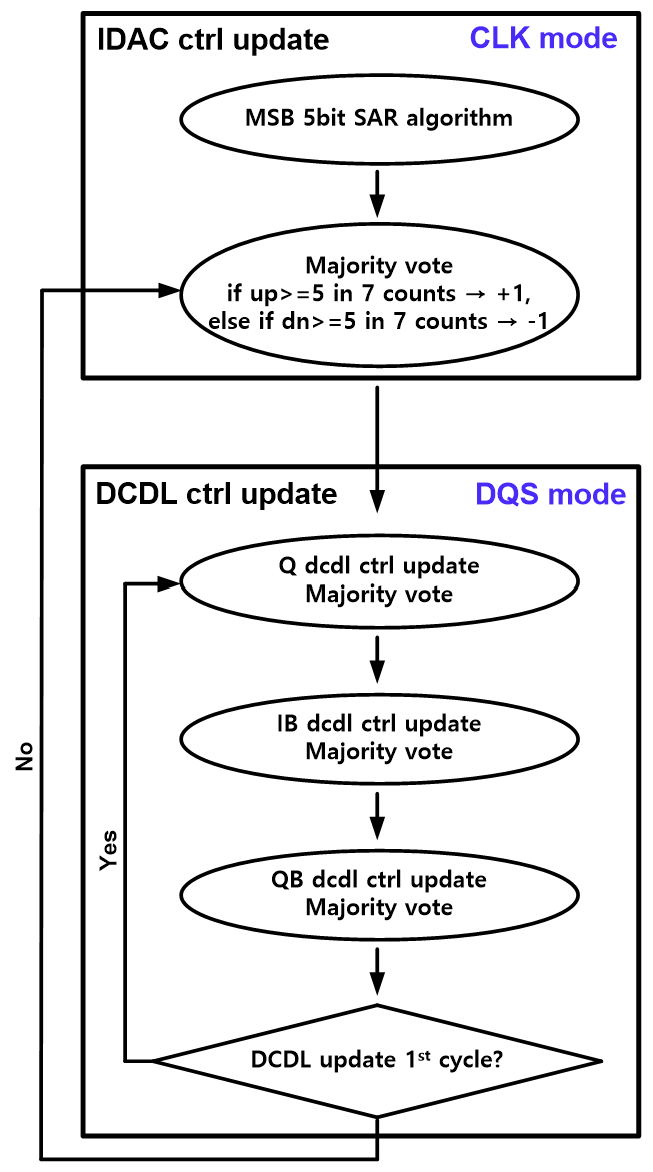## IV. SIMULATION RESULTS

A prototype of the proposed QEC is designed in a 40~nm CMOS process. The chip layout of the analog part (DCDLs, pulse generator, pulse-width detector, DIV8&3-bit counter) is shown in Fig. 12, where the area is 0.004 mm$^{2}$. The digital loop filter with an area of 0.006 mm$^{2}$ is fully synthesized, and automatically placed and routed using standard cells. The total active area of the proposed QEC is 0.01 mm$^{2}$.

##### Fig. 12. Chip layout of analog part of the proposed QEC.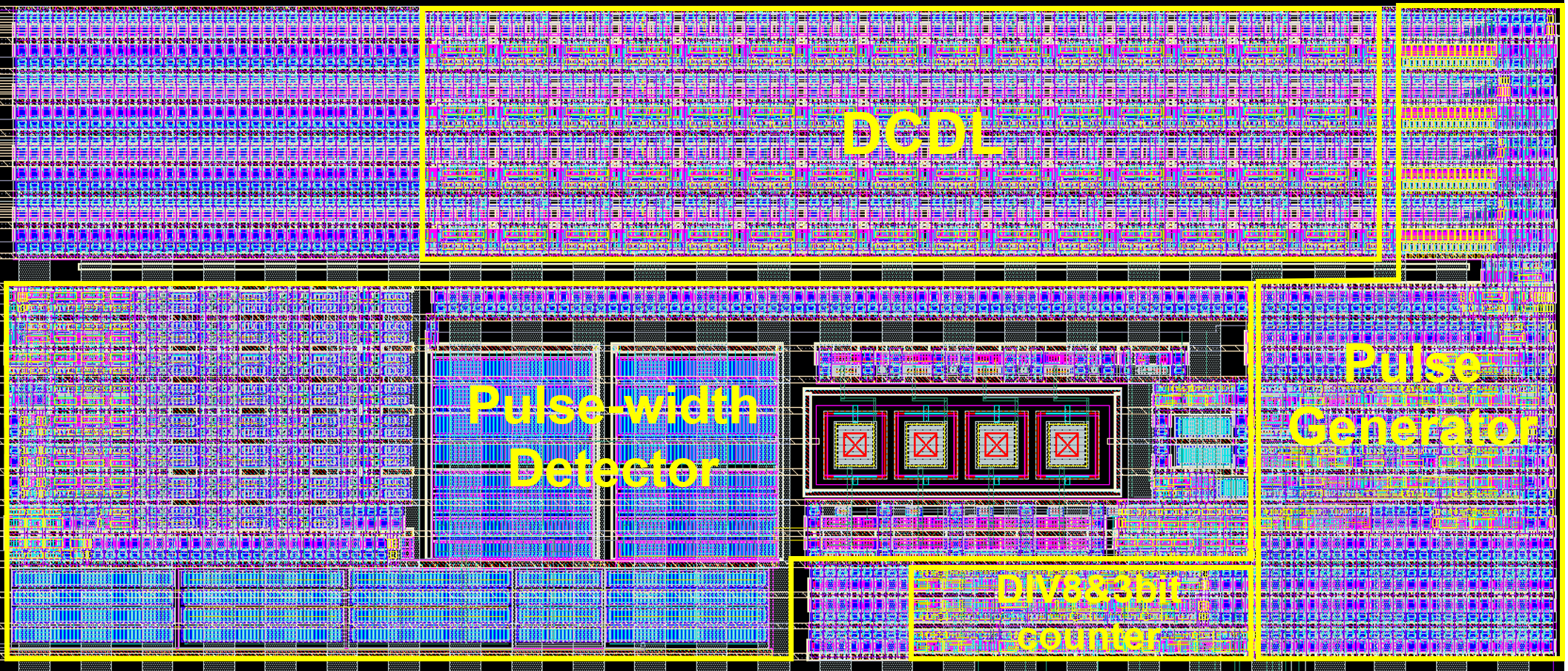The simulation is done for 1.6 GHz DQS signals in burst mode or seamless mode. The waveforms of the input quadrature DQS signals with phase errors and the corrected quadrature signals in the burst and seamless modes are depicted in Fig. 13. Fig. 13(a) and (b) illustrate the burst mode with burst lengths of 8 and 16, respectively, and Fig. 13(c) depicts the seamless mode. The simulated output phase error over a wide range of input phase error in the burst and seamless modes is presented in Fig. 14. The input correctable phase range is -43.2˚ to 43.2˚ in both modes. In the burst mode, the output phase error ranges from -5.01˚ to 1.30˚ in the correctable input phase range. In the seamless mode, the output phase error ranges from -4.16˚ to 3.85˚. The proposed circuit corrects the phase error to less than 5.01˚ for all cases.

##### Fig. 13. The waveform of input DQS signals with phase error and corrected output DQS signals (a), (b) in burst mode; (c) in seamless mode.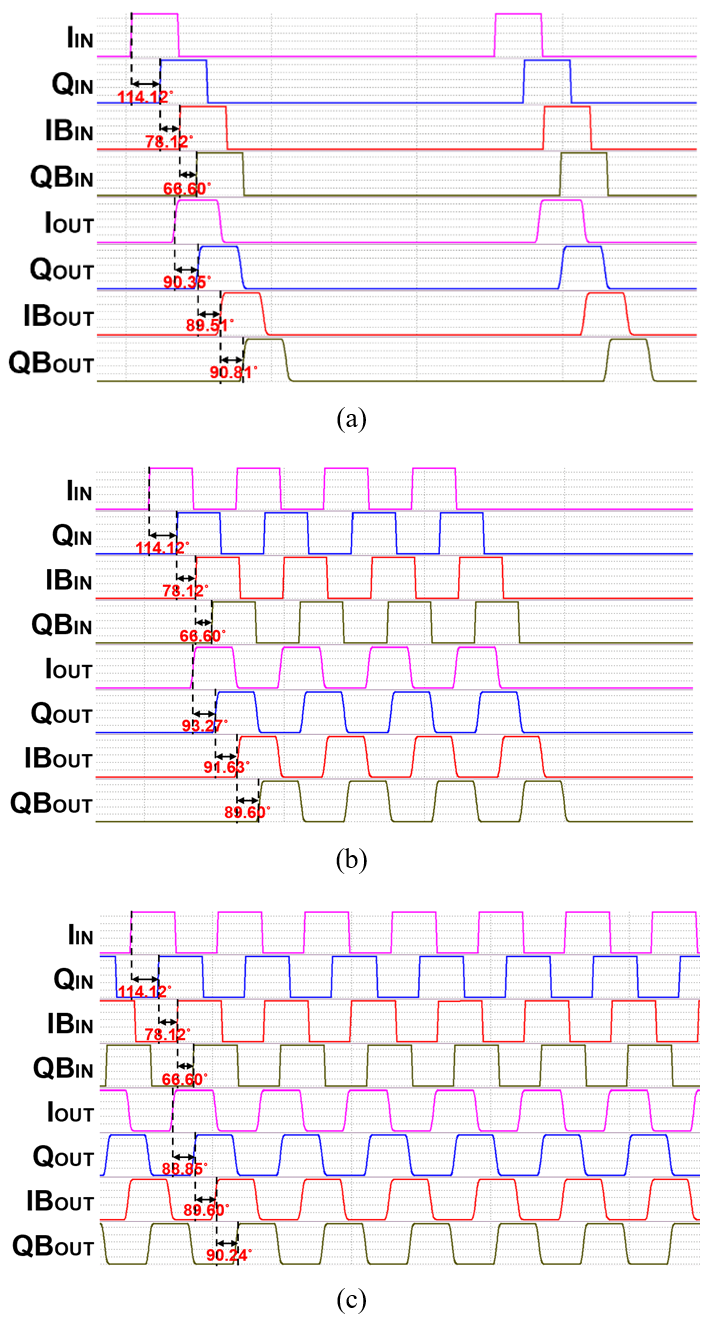##### Fig. 14. The output phase error over a wide range of input phase error in (a) burst mode; (b) seamless mode.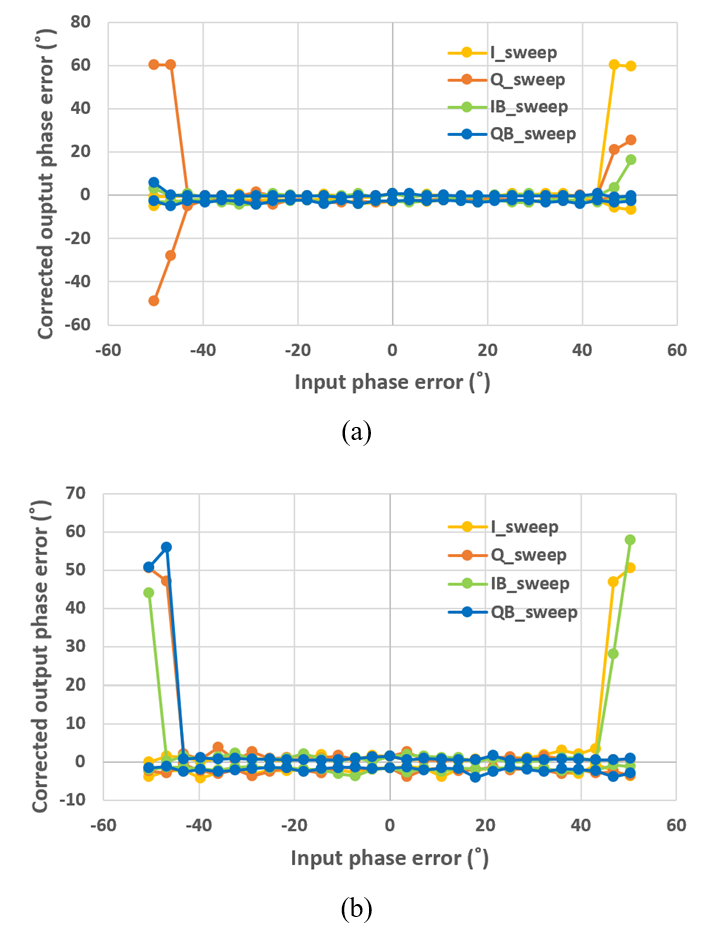The maximum correctable input phase error over the frequency of DQS signals is depicted in Fig. 15. As the frequency of the DQS signals increases, the correctable input phase error decreases because of the narrowing pulse-width of dqs_pulse, which affects the operation of the QEC.

##### Fig. 15. The maximum correctable input phase error over the frequency of DQS signals.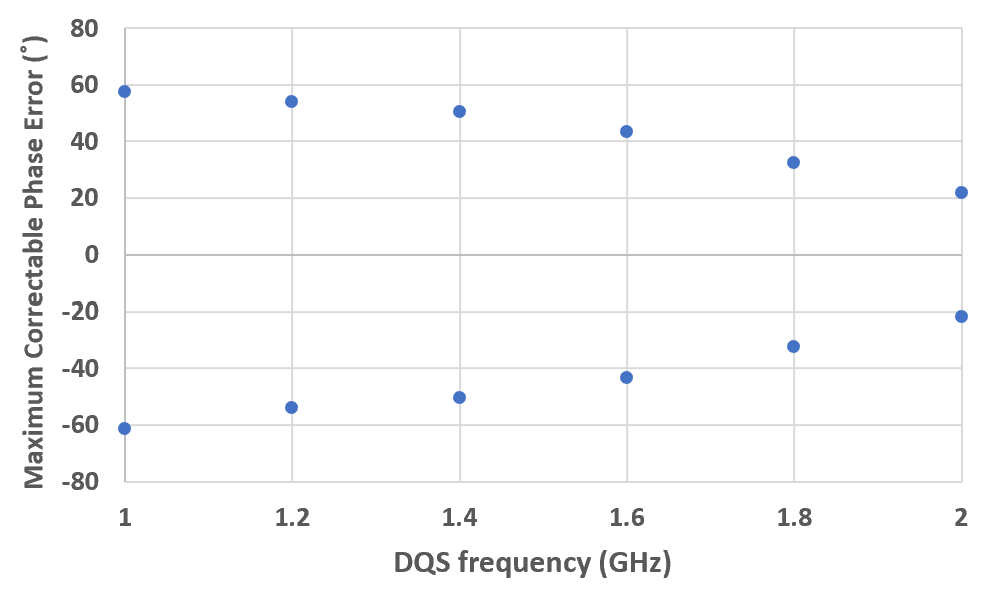The power consumption of the proposed QEC in various modes is summarized in Table I. When the QEC is off, the blocks other than the DCDLs do not operate to save power consumption and the control codes of the DCDL are fixed. In the burst mode, the power is lower because the DQS signals toggle at the lower average rates. The maximum power consumption at the frequency of 1.6 GHz is 2.42 mW in seamless mode.

The performance of the proposed quadrature error corrector is summarized and compared with the recent state-of-the-art quadrature error correctors in Table 2. The proposed QEC exhibits relatively low power consumption and a wide correctable input phase error range. The proposed QEC has the maximum phase error of 5.01$^{\circ}$ after the correction. While this phase error is larger than those with the other QECs in literature, it is still satisfactory considering that only the proposed QEC can correct the phase imbalance in the aperiodic DQS signals. In the proposed QEC, the QB signal has the worst phase error since its phase is determined by adding the estimated quarter clock period three times to the I phase. On the other hand, the other QECs assume periodicity and adjust the QB phase using the next-period's I phase as the reference, avoiding the accumulation of error.

##### Table 1. Power consumption of the proposed QEC in various modes
 seamless burst bl32 burst bl8 QEC ON DCDL + Pulse generator + DIV8&3-bit counter + Pulse-width detector 1.887 mW 1.106 mW 0.712 mW Digital Loop Filter 0.532 mW 0.438 mW 0.390 mW Total 2.419 mW 1.544 mW 1.102 mW QEC OFF DCDL + Pulse generator + DIV8&3-bit counter + Pulse-width detector 0.936 mW 0.510 mW 0.271 mW Digital Loop Filter 0.330 mW 0.329 mW 0.328 mW Total 1.266 mW 0.839 mW 0.599 mW
##### Table 2. Performance summary of the proposed QEC and comparison with recent state-of-the-art QECs
 TCAS2’17  ISSCC’20  TVLSI’19  This work Technology 40 nm 55 nm 65 nm 40 nm VDD(V) 1 1.1 1.2 1.1 Architecture Digital DLL Digital DLL Relaxation Oscillator Digital DLL + Capacitor Charging DQS correction X X X O Clock frequency (GHz) 1.25 0.8-2.3 1-3 1.0-2.0 Correctable input phase error range 8.7˚ 84.1˚ < 27˚ 86.4˚ Maximum phase error after correction 0.48˚ < 2.18˚ 1.1˚ 5.01˚ Power (mW) 2.27 8.89(cal_on)/ 3.9(cal_off) 2.08 2.42(cal_on)/ 1.26(cal_off) Area (mm$^{2}$) 0.01 0.0428 0.003 0.01 Energy consumption per unit pulse (pJ) 1.816 3.865/1.696 0.693 1.513/0.788

## V. CONCLUSIONS

This paper presents the design of a quadrature error corrector capable of correcting the phase imbalance in the aperiodic, quadrature DQS signals. The proposed QEC maintains the phase error less than 5.01˚ over a wide input phase error range of -43.2˚ to 43.2˚ and consumes the maximum power of 2.42 mW at 1.6 GHz. To the best of our knowledge, the proposed design is the only one that can be applied both to aperiodic DQS signals and periodic clock signals.

## ACKNOWLEDGMENTS

This work was supported by SK Hynix Inc. and the Inter-University Semiconductor Research Center (ISRC) of Seoul National University. The EDA Tool was supported by the IC Design Education Center.

## References

1
Oh C., et al. , Feb. 2020, A 1.1V 16GB 640GB/s HBM2E DRAM with a Data-Bus Window-Extension Technique and a Synergetic On-Die ECC Scheme, in IEEE ISSCC Dig. Tech. Papers, pp. 330-3322
Jan. 2022., High Bandwidth Memory (HBM3) DRAM, document JESD238, JEDEC Solid State Technology Association3
Kim Y., et al. , Apr. 2017, A 2.3-mW 0.01-mm$^{2}$ 1.25-GHz Quadrature Signal Corrector with 1.1-ps Error for Mobile DRAM Interface in 65-nm CMOS, IEEE Trans. Circuits Syst. II, pp. 397-4014
Shin S., et al. , Feb. 2020, A 0.8-to-2.3GHz Quadrature Error Corrector with Correctable Error Range of 101.6ps Using Minimum Total Delay Tracking and Asynchronous Calibration On-Off Scheme for DRAM Interface, in IEEE ISSCC Dig. Tech. Papers, pp. 340-3425
Chae J., et al. , Apr. 2019, A Quadrature Clock Corrector for DRAM Interfaces, with a Duty-Cycle and Quadrature Phase Detector Based on a Relaxation Oscillator, IEEE Trans. Very Large Scale Integr. Syst., pp. 978-9826
Ko H., et al. , Feb. 2020, A 3.2-GHz Quadrature Error Corrector for DRAM Transmitters, Using Replica Serializers and Pulse-Shrinking Delay Lines, IEEE Solid-State Circuits Letters, pp. 38-41Seo-Yeong Jo received the B.S. and M.S. degree in electrical and computer engineering from Seoul National University, Seoul, Korea, in 2020 and 2022, respectively. Her research interests include low-power mixed-signal systems and high-speed I/O circuits.

Jinhyung Lee received the B.S. and Ph.D. degrees in electrical engineering and computer science from Seoul National University, Seoul, South Korea, in 2014 and 2019, respectively. He joined SK Hynix, Icheon, South Korea. His current research interests include high-speed I/O circuits, adaptive equalizers, and high bandwidth memory (HBM).

Myeong-Jae Park received the B.S., M.S., and Ph.D. degrees from Seoul National University, Seoul, South Korea, in 2003, 2006, and 2014, respectively. From 2004 to 2009, he was a Design Engineer with Anapass, South Korea, involved in LCD intrapanel interfaces. During his graduate studies, he held a summer position with Rambus Inc., in 2012, involved in ultra high-speed serial interface. Since 2014, he has been a Senior Research Engineer with SK Hynix, Icheon, South Korea. He is currently the Inventor of the Advanced Intra-Panel Interface, an intra-panel interface standard of Samsung’s flat panel TVs. His research interests include low-power mixed-signal systems, power electronics, and their design methodologies.

Deog-Kyoon Jeong received the B.S. and M.S. degrees in electronics engineering from Seoul National University, Seoul, South Korea, in 1981 and 1984, respectively, and the Ph.D. degree in electrical engineering and computer sciences from the University of California at Berkeley, Berkeley, CA, USA, in 1989. From 1989 to 1991, he was with Texas Instruments, Dallas, TX, USA, as a Member of the Technical Staff and worked on the modeling and design of Bipolar CMOS (BiCMOS) gates and the single-chip implementation of the Scalable Processor ARChitecture (SPARC). He joined the Faculty of the Department of Electronics Engineering and InterUniversity Semiconductor Research Center, Seoul National University, where he is currently a Professor. He was one of the cofounders of Silicon Image (now Lattice Semiconductor), Sunnyvale, CA, USA, which specialized in digital interface circuits for video displays, such as digital visual interface (DVI) and high definition multimedia interface (HDMI). His main research interests include the design of high-speed I/O circuits, phase-locked loops (PLLs), and memory system architecture.

Jaeha Kim (S’95-M’03-SM’09) received the B.S. degree in electrical engineering from Seoul National University, Seoul, South Korea, in 1997, and the M.S. and Ph.D. degrees in electrical engineering from Stanford University, Stanford, CA, USA, in 1999 and 2003, respectively. He is currently an Associate Professor with Seoul National University. Prior to joining Seoul National University in 2010, he was with Stanford University as an Acting Assistant Professor from 2009 to 2010, with Rambus, Inc., Los Altos, CA, USA, as a Principal Engineer from 2006 to 2009, and with the Inter-university Semiconductor Research Center (ISRC), Seoul National University, as a Postdoctoral Researcher from 2003 to 2006. From 2001 to 2003, he was with True Circuits, Inc., Los Altos, CA, USA, as a Circuit Designer. In 2015, he founded Scientific Analog, Inc., Palo Alto, CA, USA, an EDA company involved in analog/mixed-signal verification. His research interests include low-power mixed-signal systems and their design methodologies. Dr. Kim served on the Technical Program Committees of the International Solid-State Circuits Conference (ISSCC), Custom Integrated Circuits Conference (CICC), Design Automation Conference (DAC), International Conference on Computer Aided Design (ICCAD), and Asian Solid-State Circuit Conference (ASSCC). He was cited as a Top 100 Technology Leader of Korea in 2020 by the National Academy of Engineering of Korea (NAEK). He was a recipient of the Takuo Sugano Award for Outstanding Far-East Paper at the 2005 International Solid-State Circuits Conference (ISSCC) and the Low Power Design Contest Award at the 2001 International Symposium on Low Power Electronics and Design (ISLPED).